#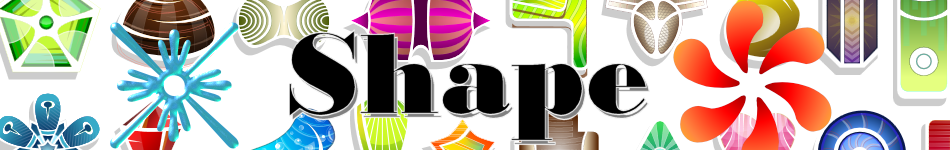There are 366 different Starters of The Day, many to choose from. You will find below some starters on the topic of Shape. A lesson starter does not have to be on the same topic as the main part of the lesson or the topic of the previous lesson. It is often very useful to revise or explore other concepts by using a starter based on a totally different area of Mathematics.

Main Page

### Shape Starters: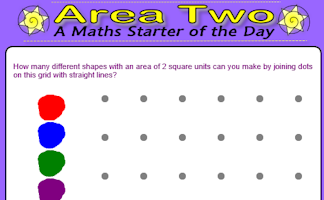How many different shapes with an area of 2 square units can you make by joining dots on this grid with straight lines?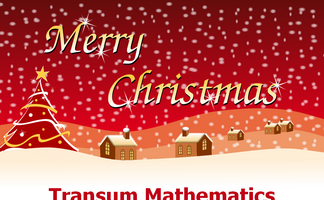Which of the two shapes has the largest area? You will be surprised!Find the loci of the goat's position as it eats the grass while tethered to the rope.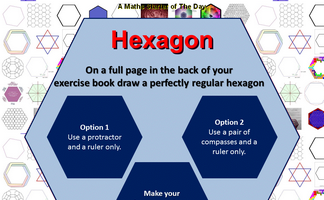On a full page in the back of your exercise book draw a perfectly regular hexagon.How many rectangles can you find in this pattern? Can you come up with a systematic method for counting them all?Work out how many squares are there altogether in the given pattern then tackle the chess board.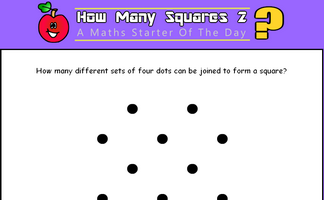How many squares can be found by joining four dots on the grid?How many Triangles can you find in the diagram?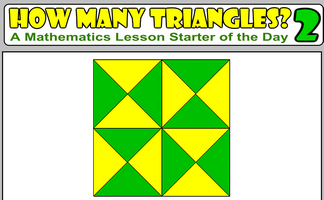How many triangles are hidden in the pattern? What strategy might you use to count them all to ensure you don't miss any out?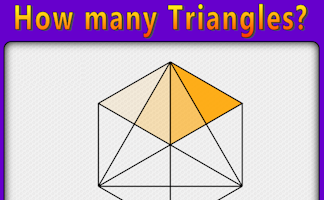Find a systematic way of counting the number of triangles in the given diagram.Memorise a picture made up of geometrical shapes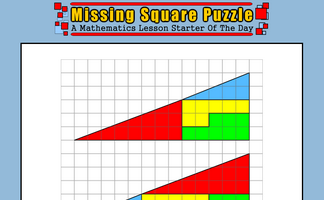The missing square puzzle is an optical illusion used to help students reason about geometrical figures.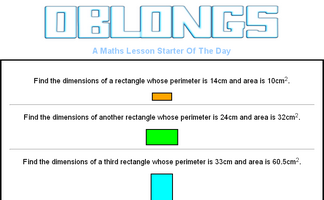Find the dimensions of a rectangle given the perimeter and area.If two squares overlap, what shapes can the overlapping region make?On a full page in the back of your exercise book draw a perfectly regular pentagon.Solve the riddle to find the name of the polygon then sum the interior angles.A "My first is in..." type riddle leading to a polygon interior angle calculation.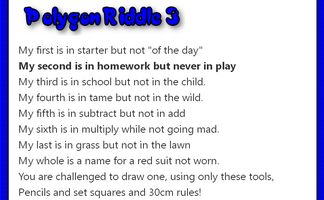A 'My first is in...' riddle that describes a geometrical shape. Can you construct it?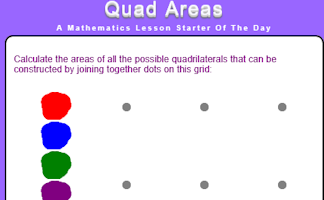Calculate the areas of all the possible quadrilaterals that can be constructed by joining together dots on this grid.Can you draw 4 straight lines, without taking your pencil off the paper, which pass through all 9 roses?Find a trapezium, a triangle and a quadrilateral where all of the angles are square numbers.Write down the names of all the mathematical shapes you know.Separate three rows of three animals using three squares.Using six pencils can you make two equal sized squares?

## Exercises#### Angle Parallels

Understand and use the relationship between parallel lines and alternate and corresponding angles.#### Angles in a Triangle

A self marking exercise involving calculating the unknown angle in a triangle.#### Area and Perimeter

Show that you know the area and perimeter formulas of basic shapes.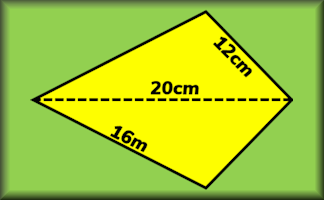#### Area and Perimeter of a Kite

A short exercise to practise using the formulae for area and perimeter of a kite.#### Area and Perimeter of a Parallelogram

Many different ways to practise your skills finding the areas and perimeters of parallelograms.#### Area and Perimeter of a Rectangle

Questions on the areas and perimeters of rectangles which will test your problem solving abilities.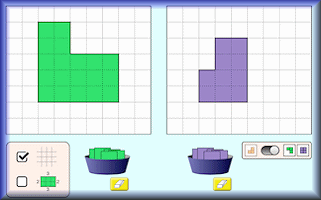#### Area Builder

An interactive workspace in which to make shapes using square tiles with given areas and perimeters.#### Area Maze

Use your knowledge of rectangle areas to calculate the missing measurement of these composite diagrams.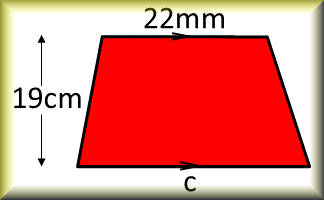#### Area of a Trapezium

Check that you can find the area of a trapezium and use the trapezium area formula for problem solving.#### Area of a Triangle

Calculate the areas of the given triangles in this self marking quiz.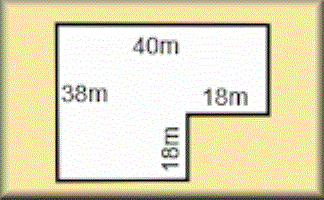#### Areas of Composite Shapes

Find the areas of combined (composite) shapes made up of one or more simple polygons and circles.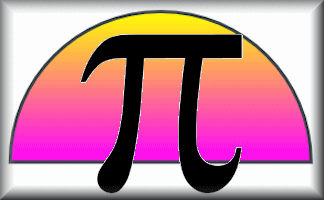#### Circles

Practise using pi to calculate various circle measurements. There are six levels of difficulty.#### Congruent Pairs

Match the congruent shapes, those with the same shape and size.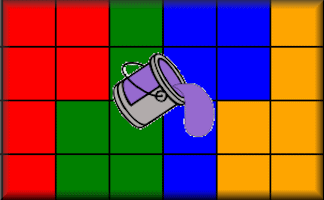#### Congruent Parts

Use the colours to dissect the outlines into congruent parts.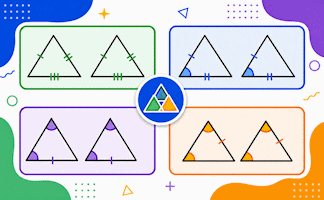#### Congruent Triangles

Test your understanding of the criteria for congruence of triangles with this self-marking quiz.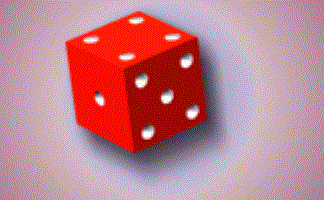#### Faces, Edges and Vertices

Calculate the number of faces, edges and vertices on 3D Shapes.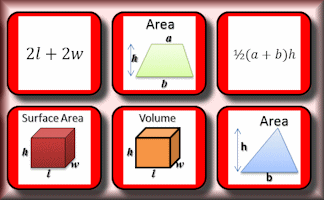#### Formulae Pairs

Find the matching pairs of diagrams and formulae for basic geometrical shapes.#### Fractal Mosaic

Follow the precise instructions to create the ever-growing fractal mosaic pattern.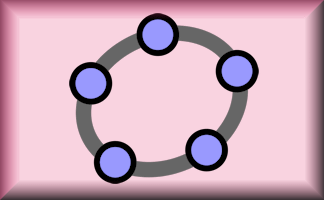#### Geometry Toolbox

Create your own dynamic geometrical diagrams using this truly amazing tool from GeoGebra.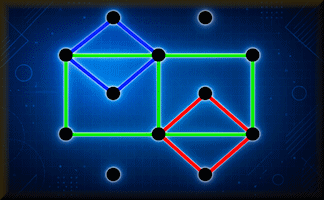#### How Many Squares? 2

How many different sets of four dots can be joined to form a square?#### How Many Triangles?

A self marking step by step approach to calculating the number of triangles in a design.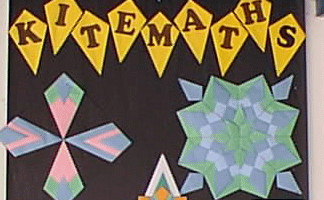#### Kite Maths

Can you make a kite shape from a single A4 size sheet of paper using only three folds?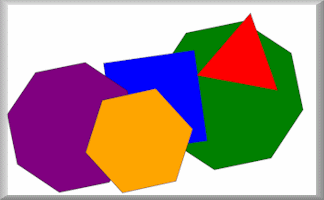#### Mathterpieces

Memorise eight pictures made up of geometrical shapes then sort them into order.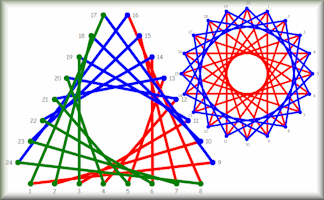#### Online Curve Stitching

Create curves with straight lines using this online alternative to needle and thread.#### Online Logo

An online version of the Logo programming language with 30 mathematical challenges.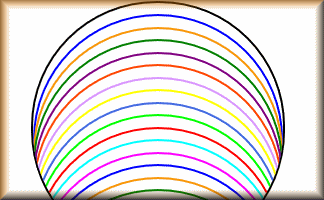#### Optical Illusions

Don't let your brain be fooled by these geometric optical illusions in this online quiz.#### Pin Board

Rows and columns of dots that can be joined using straight lines to create shapes.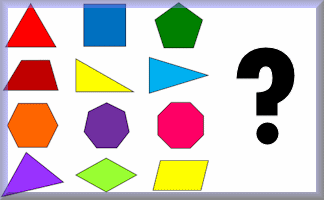#### Polybragging

The Transum version of the Top Trumps game played online with the properties of polygons.#### Polygon Angles

A mixture of problems related to calculating the interior and exterior angles of polygons.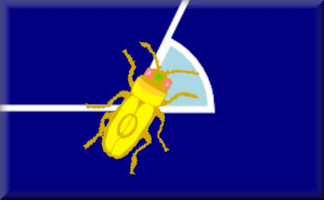#### Polygon Angles Animation

Barbara Bug walks around a regular hexagon turning through each of the exterior angles as she goes.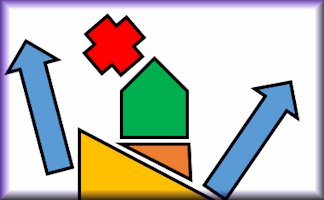#### Polygon People

Name the polygons and other geometrical shapes that make up the Polygon People.#### Polygon Pieces

Arrange the nine pieces of the puzzle on the grid to make different polygons.#### Polygon Properties

Connect the names of the polygons with the descriptions of their properties.#### Polygons

Name the polygons and show the number of lines and order of rotational symmetry.Calculate the areas of all the possible quadrilaterals that can be constructed by joining together dots on this grid.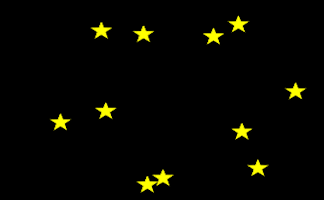#### Shapes In The Stars

Join up the stars to find the hidden regular polygons.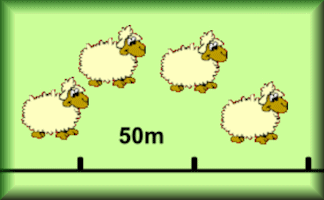#### Sheep Herding

Arrange the sheep in the field according to the instructions. An introduction to loci.#### Similar Parts

Use the colours to dissect the outlines into similar parts.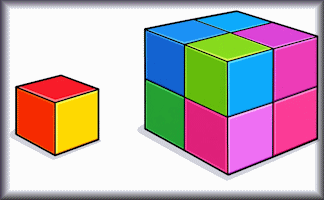#### Similar Shapes

Questions about the scale factors of lengths, areas and volumes of similar shapes.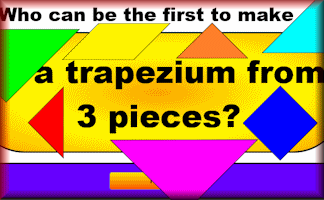#### Tangram Challenge

A series of tangram challenges in increasing order of difficulty.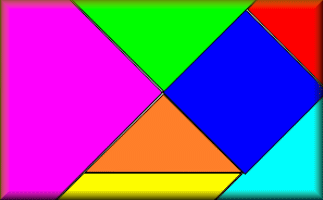#### Tangram Table

Use the pieces of the tangram puzzle to make the basic shapes then complete the table showing which shapes are possible.#### Tantrum

A game, a puzzle and a challenge involving counters being placed at the corners of a square on a grid.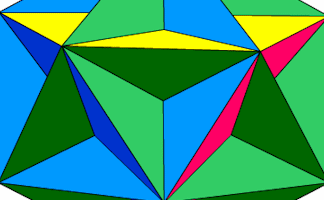#### The Great Dodecahedron

Pupils are not allowed to use their hands to point but must describe fully any shapes they can see in this picture.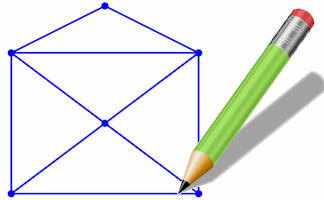#### Without Lifting The Pencil

Can you draw these diagrams without lifting your pencil from the paper? This is an interactive version of the traditional puzzle.#### Xmas Ornaments

A hands on activity requiring students to arrange Christmas ornaments in a square box.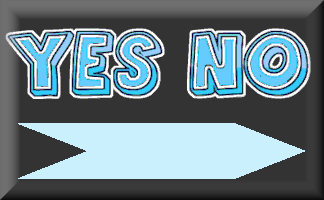#### Yes No Questions

A game to determine the mathematical item by asking questions that can only be answered yes or no.

### Search

The activity you are looking for may have been classified in a different way from the way you were expecting. You can search the whole of Transum Maths by using the box below.

Have today's Starter of the Day as your default homepage. Copy the URL below then select
Tools > Internet Options (Internet Explorer) then paste the URL into the homepage field.

Set as your homepage (if you are using Internet Explorer)

Do you have any comments? It is always useful to receive feedback and helps make this free resource even more useful for those learning Mathematics anywhere in the world. Click here to enter your comments.For All: